Hypertetrahedron
 What is the Hypertetrahedron? Tetrahedrons in Perspective  Characteristics of the Hypertetrahedron Projections Nets Formulas Hypertetrahedron on the Internet References.
 To the Main Page     "Mathematische Basteleien"

What is the Hypertetrahedron?
The hypertetrahedron is the four-dimensional tetrahedron. Other names are simplex or pentatope.

Tetrahedrons in Perspective top
The four-dimensional tetrahedron belongs to the world of thoughts. You can approach it through analogy considerations. You go from the tetrahedron to the hypertetrahedron. This procedure is legitimized by the "permanent principle", which is often used in mathematics to get from the known to the unknown.
 .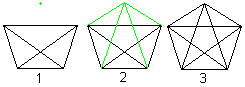.. If you connect the corners of a tetrahedron (1) with a fifth point (2), then you get the perspective view of a hypertetrahedron (3).
While a quadrilateral with the two diagonals can be seen as a perspective view of a tetrahedron, a pentagon with the diagonals represents a model of the hypertetrahedron.

Characteristics of the Hypertetrahedron   top
The hypertetrahedron has 5 corners (1 tetrahedron and the fifth point) and 10 edges (1 tetrahedron with 6 edges and 4 connecting lines to the fifth point).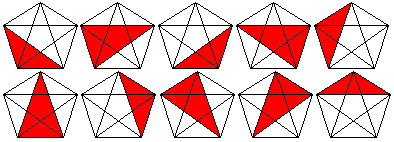The hypertetrahedron has 10 triangles.The tetrahedron is covered by four triangles. In the same way five tetrahedrons form the hypertetrahedron.

If you know the 3D-view, you can look at the hypertetrahedron three-dimensionally, too.4 tetrahedrons, 6 triangles and 4 edges meet at each corner.
3 tetrahedrons and 3 triangles meet at each edge.
2 tetrahedrons meet at each triangle.

Projections    top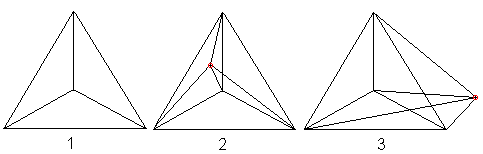If you give the top view of a tetrahedron (=central projection) (1) and a fifth point (red) inside (2) or outside (3), you get two models of the hypertetrahedron. All points must have the same distances from each other in "reality".

The hypertetrahedron 2 is a triangle with certain lines inside, the hypertetrahedron 3 a quadrilateral. The model above is a pentagon.

You recognize in all models of the hypertetrahedrons, that four edges meet at each corner.

Nets    top
 ...... If you spread out the tetrahedron, you get its net. Together the four triangles have 4*3=12 sides. 2*3=6 sides (red) are bound. If you build a tetrahedron, you must stick the remaining 6 sides in pairs.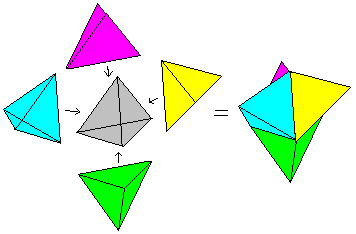... If you spread out the hypertetrahedron, you get 5 tetrahedrons as its net. Together the five tetrahedrons have 5*4=20  triangles. 2*4=8 triangles are bound. If you build a hypertetrahedron, you must stick the remaining 12 triangles in pairs.

Formulas   top
The considerations above get a safer foundation by formulas.

"Tetrahedrons" with the dimensions 1, 2 and 3 have the following characteristics.You would have to determine the data of the hypertetrahedron in the last line. Dimension=4, corners=5 is clear. The following law describes how the sequences of the edges and triangles must be continued.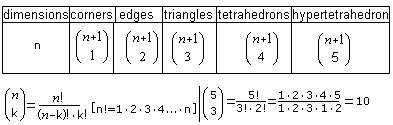If you give n=4 into the terms of the chart, then you get the following data for the hypertetrahedron.The results for the 5-dimensional tetrahedron are:Hypertetrahedron on the Internet      top

German

Marco Möller
Polytope

Wikipedia
Pentachoron

English

Eric W. Weisstein, (MathWorld)
Pentatope, Simplex

Paul Bourke
HyperSpace, User Manual

Wikipedia
Pentatope

References    top
(1) Fritz Reinhardt, Heinrich Soeder: dtv-Atlas zur Mathematik I (Seite 172), München 1977

Feedback: Email address on my main page